Author: Oscar Cronquist Article last updated on July 31, 2021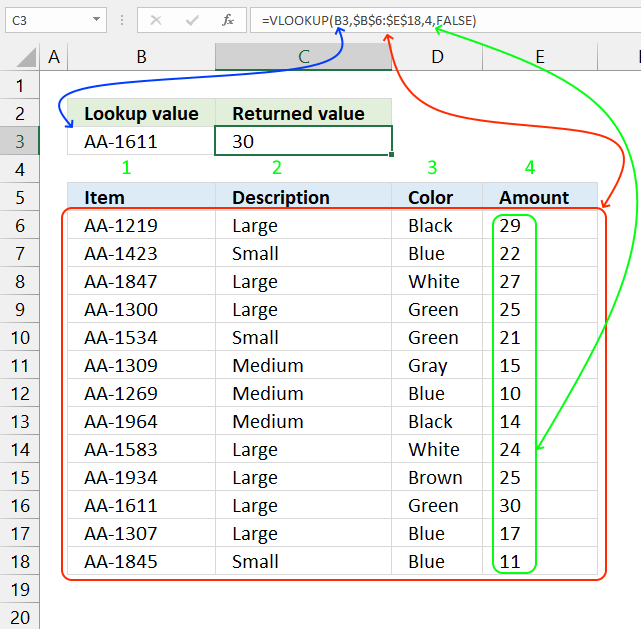The VLOOKUP function lets you search the leftmost column for a value and return another value on the same row in a column you specify.

Use the new XLOOKUP function which is an improved version of the VLOOKUP function available for Excel 365 users.

VLOOKUP stands for vertical lookup and your data (table_array) must be organized into records, a record on each row. To search records excel must look vertically in the data array. HLOOKUP stands for horizontal lookup but that is for another post.

Formula in cell C3:

=VLOOKUP(B3, B6:E18, 4, FALSE)

The VLOOKUP function uses lookup value AA-1611 in cell B3 and searches Table1 (cell range B6:E18) in the leftmost column. A matching value is found on row 16.

The third argument is which column you want to fetch the corresponding value from. In this example, column 4 is entered and £30.00 is returned in cell C3.

The fourth and last argument lets you choose between approximate and exact match, in this case, FALSE meaning EXACT match.

The VLOOKUP function is designed to return only one value, however, there are workarounds to fetch all matching values.
5 easy ways to VLOOKUP and return multiple values
I recommend the FILTER function if you are an Excel 365 user.

### Excel function syntax

VLOOKUP(lookup_value, table_array, col_index_num, [range_lookup])

### Arguments

 lookup_value Value you want to lookup. table_array The range you want to use, remember that the VLOOKUP function always looks in the leftmost column in your specified range. col_index_num The column number which contains the return value. [range_lookup] True or False (boolean value). True - approximate match, the leftmost column must be sorted ascending. False - Exact match.

## 1. VLOOKUP - approximate match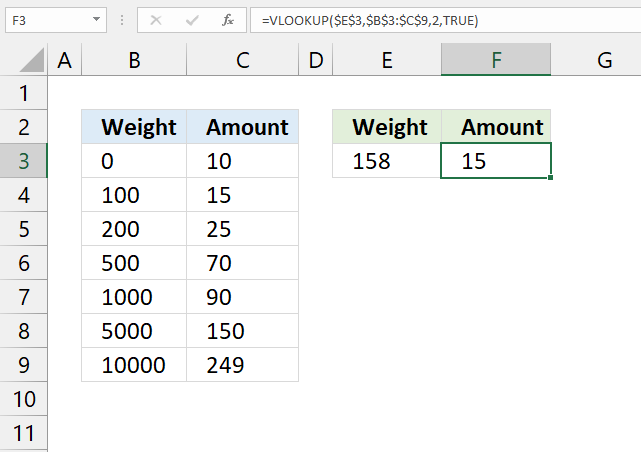The fourth argument [range_lookup] allows you to choose if you want an APPROXIMATE or EXACT match.

You want, in most cases, to use the EXACT match however the default value is an APPROXIMATE match. So make sure you know what you are doing.

True - Approximate match (default)
False - Exact match

This means that you get the approximate match if you don't specify the fourth argument at all.

VLOOKUP(lookup_value, table_array, col_index_num, [range_lookup])

The example above shows you the difference between EXACT and APPROXIMATE match. You must have your leftmost column sorted in an ascending order or you may get incorrect results.

The approximate match finds the largest value that is less than or equal to the lookup_value.

The approximate match is useful if you want to find which group or range your value belongs to. The example above shows that value 100 is the largest value that is less than or equal to 158, the corresponding value to 100 in column C is 15.

Formula in cell C3:

=VLOOKUP(E3, B3:C9, 2, TRUE)

Example weights and result amounts:

 Weight Amount 0 10 50 10 99 10 100 15 101 15 150 15 199 15 200 25 201 25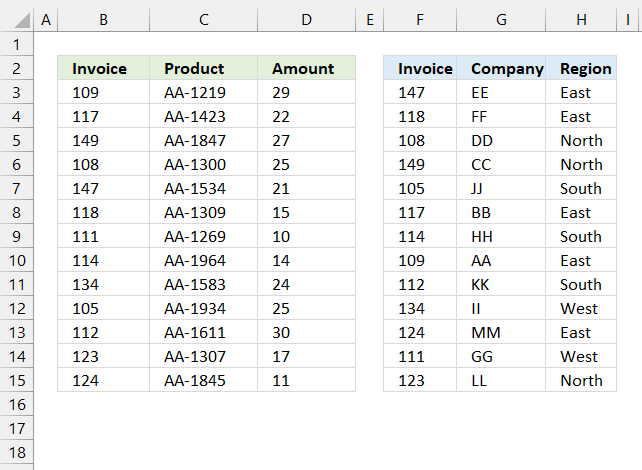A Pivot Table can't work with related tables (power pivots do) however the VLOOKUP function can quickly merge related tables so you then can analyze data in a Pivot Table.

These two tables are related meaning they share a column (invoice), you can use the following formula to merge these two data sets based on the invoice column, this will organize data and put corresponding data next to the appropriate invoice number.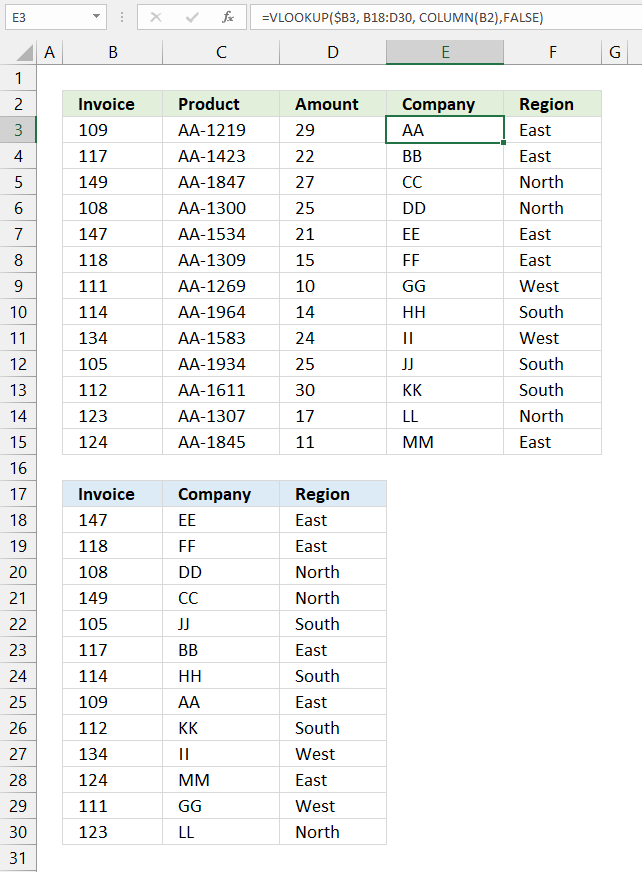The image above shows both data sets and the VLOOKUP function in cell E3.

Formula in cell E3:

=VLOOKUP(\$B3, B18:D30, COLUMN(B2), FALSE)

Copy formula in cell E3 and paste to E3:F15.

### Explaining formula in cell E3

#### Step 1 - Calculate column number to get values from

The COLUMN function returns a number representing the position of a column counting from left to right based on a cell reference.

We can use this to create a dynamic number that changes when we copy the formula and paste to adjacent cells.

The technique that makes this possible is a relative cell reference, in this case, cell reference B2.

COLUMN(B2) returns 2.

#### Step 2 - Change cell reference to a relative cell reference

We want to use values only from column B, use the dollar sign to lock the cell reference to column B.

This keeps the cell reference pointing to column B, however, the row part of the cell reference is relative meaning it will change when the formula is copied and pasted to other cells.

\$B3

#### Step 3 - Get value

VLOOKUP(\$B3, B18:D30, COLUMN(B2), FALSE)

becomes

VLOOKUP(109, {147, "EE", "East";118, "FF", "East";108, "DD", "North";149, "CC", "North";105, "JJ", "South";117, "BB", "East";114, "HH", "South";109, "AA", "East";112, "KK", "South";134, "II", "West";124, "MM", "East";111, "GG", "West";123, "LL", "North"}, {2}, FALSE)

and returns "AA"

## 3. How to use the VLOOKUP function with a cell reference specified in a cell?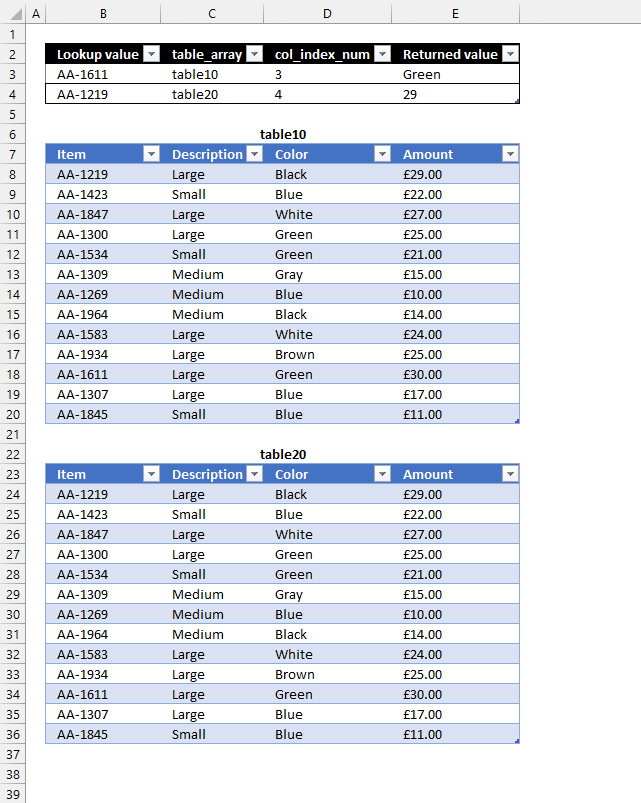This example shows you how to use the VLOOKUP function with multiple tables, it searches an Excel Table based on the specified table name in cell C3. The image above shows two Excel tables, table10 and table20.

Here is how it works, the lookup value is in B3, the Excel Table name is in C3, the column number in cell D3, and the formula is in cell E3.

The formula in cell E3 uses the values in B3, C3, and D3 to extract the appropriate value, this example shows how to use cell values instead of hardcoded values as arguments.

Formula in cell E3:

=VLOOKUP(B3,INDIRECT(C3),D3,FALSE)

### Explaining formula in cell E3

#### Step 1 - Convert text string to a cell reference

The INDIRECT function allows you to convert a string to a valid cell reference.

INDIRECT(C3)

becomes

INDIRECT("table10")

and returns table10.

#### Step 2 - Get value

VLOOKUP(B3, INDIRECT(C3), D3, FALSE)

becomes

VLOOKUP("AA-1611", table10, 3, FALSE)

and returns "Green" in cell E3.

The INDIRECT function lets you use a cell value as the table_array argument, this lets you quickly change the value in cell C3 and search multiple tables.

## 4. What if the lookup value is not in the leftmost column?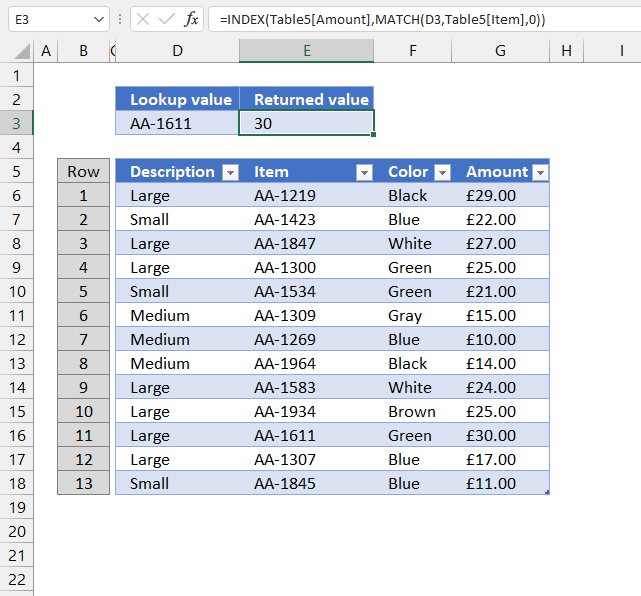The disadvantage with the VLOOKUP function is that it can only look for a value in the leftmost column. If you don't have your values in the leftmost column you have three options, rearrange your table, use the XLOOKUP function (Excel 365), or use another method demonstrated below.

The INDEX and MATCH function allows you to search any column in a table and return a value in any column on the same row. This formula is so versatile that I actually prefer INDEX + MATCH over the VLOOKUP function.

Formula in cell C3:

=INDEX(G6:G18, MATCH(D3, E6:E18, 0))

### Explaining formula in cell C3

#### Step 1 - Find the relative position of a lookup value

The MATCH function lets you look for a value in a column and return the relative position of the found value in the array.

MATCH(D3, E6:E18, 0)

becomes

MATCH("AA-1611", E6:E18, 0)

and returns 11. AA-1611 is found on row 11 in cell range E6:E18.

#### Step 2 - Get value

The INDEX function returns a value from a cell range, you specify which value based on a row and column number.

INDEX(array, [row_num], [column_num])

INDEX(G6:G18, MATCH(D3, E6:E18, 0))

becomes

INDEX(G6:G18, 11)

and returns 30 in cell C3.

## 5. How to handle VLOOKUP errorsThe IFERROR function allows you to return a value if the VLOOKUP function returns an error, in this case NOT FOUND!

## 6. How to use VLOOKUP in VBA?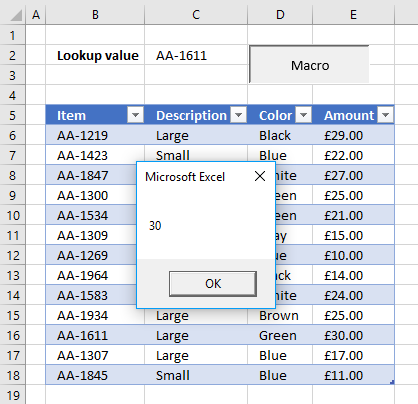The following VBA code demonstrates how to use VLOOKUP in a macro.

The macro searches B6:B18 using the value in cell C2 and displays the result in a message box.

```VBA code
Sub VLP()
MsgBox Application.WorksheetFunction.VLookup(Range("C2"), Range("B6:E18"), 4, False)
End Sub
```